Courses

# RD Sharma Solutions - Ex-18.2, Symmetry, Class 7, Math Class 7 Notes | EduRev

## Class 7 : RD Sharma Solutions - Ex-18.2, Symmetry, Class 7, Math Class 7 Notes | EduRev

The document RD Sharma Solutions - Ex-18.2, Symmetry, Class 7, Math Class 7 Notes | EduRev is a part of the Class 7 Course RD Sharma Solutions for Class 7 Mathematics.
All you need of Class 7 at this link: Class 7

#### Question 1:

In the following figures, the mirror line (i.e. the line of symmetry) is given as dotted line. Complete each figure performing reflection in the dotted (mirror) line. Also, try to recall the name of the complete figure.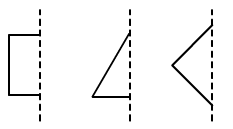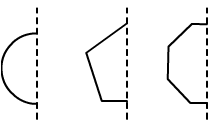(a) It will be a square.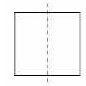(b) It will be a triangle.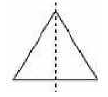(c) It will be a rhombus.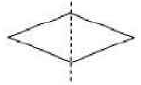(d) It will be a circle.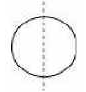(e) It will be a pentagon.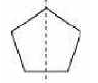(f) It will be an octagon.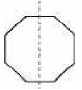Question 2:

Each of the following figures shows paper cuttings with punched holes. Copy these figures on a plane sheet and mark the axis of symmetry so that if the paper is folded along it, then the wholes on one side of it coincide with the holes on the other side.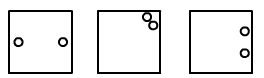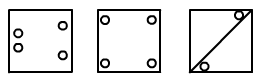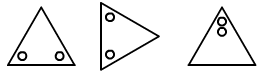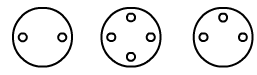The lines of symmetry in the given figures are as follows:
(a)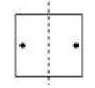(b)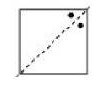(c)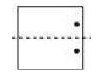(d)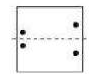(e)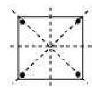(f)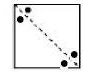(g)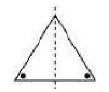(h)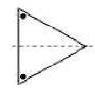(i)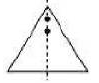(j)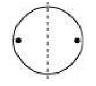(k)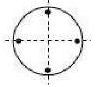(l)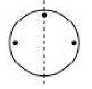#### Question 3:

In the following figures if the dotted lines represent the lines of symmetry, find the other hole (s).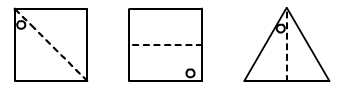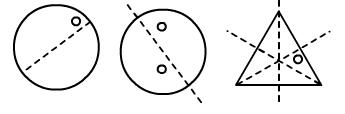The other holes in the given figures are as follows:
(a)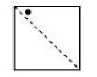(b)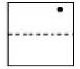(c)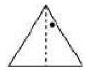(d)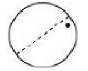(e)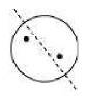(f)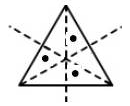Offer running on EduRev: Apply code STAYHOME200 to get INR 200 off on our premium plan EduRev Infinity!

97 docs

,

,

,

,

,

,

,

,

,

,

,

,

,

,

,

,

,

,

,

,

,

,

,

,

,

,

,

,

,

,

;